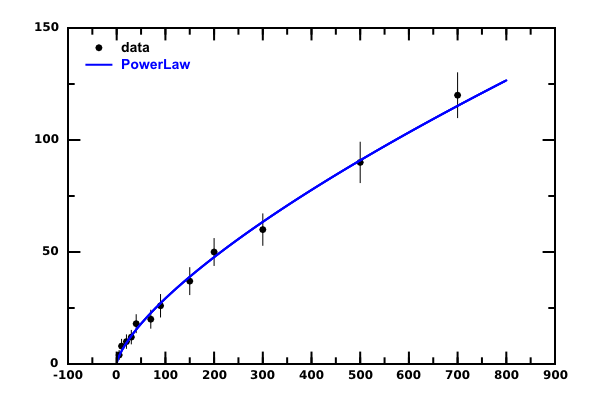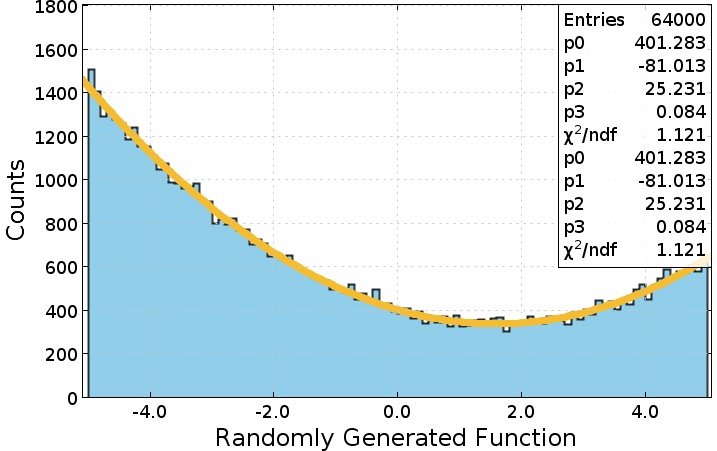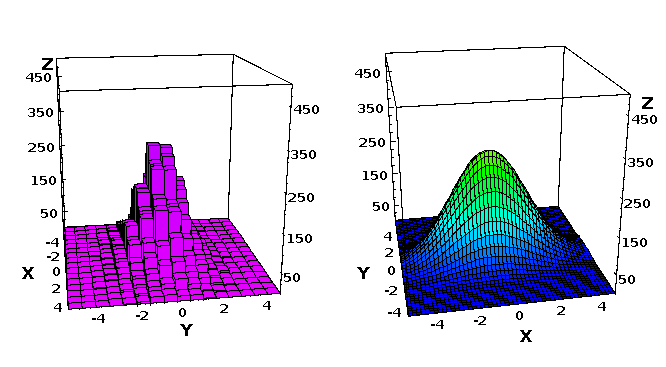Limitted access. First login to DataMelt if you are a full DataMelt member. Then login to HandWiki as a user.

This section deals with advanced techniques of fitting data using data in arbitrary dimension, arbitrary function. In this section we will discuss how to perform more interactive fit using scripting.

## Chi2 fit in 1D

Let us perform a fit of data points using a function defined by a Python script:

from java.lang.Math import *
from jhplot import *
class PowerLawFunc():         # function describing a power law
def __init__(self, p0,p1):
self.p0=p0;   self.p1=p1;
def valueOf(self, x):
return  self.p0*pow(x,self.p1); # power law
def getPlot(self,min,max): # plotting part
f=P1D("p0*x^p1")
step=(max-min)/100.0
for i in range(100):
x=min+step*i
y=self.valueOf(x)
return f

As you can see, this is a simple power-law function of one variable "x" defined in the method "valueOf". One interesting method of this function is getPlot(min,max) that allows to plot this function between min and max. This method returnsjhplot.P1D plottable onjhplot.HPlot

Now let us perform a fit with this function using Minuit minimisation program and the Chi2 method.

$\displaystyle{ \chi^2 = \sum_{i} \frac{(y_i - e_i)^2}{ (\sigma_i)^2} }$

where "y" is the data point, "e" is the expectation, $\sigma$ is “variance” related to the measurement error for "y"

Let us make a Jython script which:

1. Performs Chi2 fit with the above function
2. Prints evolution of chi2 for each iteration and print final chi2
3. Calculates Minuit convergence steps and change Migrad/Minuit strategy in case of a failed fit
4. Calculates calculates Migrad parameter correlations
5. Calculate uncertainty on the fit parameters
6. Plot data and the minimized function

For this task, we will useorg.freehep.math.minuit.MnMigrad class. The script that does all the above using this class is shown bellow:

The fit result of the data is shown below, where the blue result is the fit:The fit parameters are:

p0=1.14152  +/-  0.276695
p1=0.704415 +/-  0.0431544

Here is the complete output of the script:

start Migrad
Chi2= 470.358172895
Chi2= 151.491535555
Chi2= 152.13739461
Chi2= 151.491535555
Chi2= 150.199077966
Chi2= 146.963922788
Chi2= 137.231555692
Chi2= 108.020960351
Chi2= 29.5665676492
Chi2= 224.944087841
Chi2= 11.1675109612
Chi2= 3.09063261351
Chi2= 2.83286407423
Chi2= 2.83089798953
Chi2= 2.60969185498
Chi2= 2.58038208008
Chi2= 2.37208952261
Chi2= 2.37208949274
Chi2= 2.37209280753
Minuit did successfully converge.
# of function calls: 77
minimum function value: 2.37209
minimum edm: 2.72672e-09
minimum internal state vector: LAVector parameters: 1.14152 0.704415
minimum internal covariance matrix: LASymMatrix parameters:
0.0765603 -0.0117485
-0.0117485 0.00186230

# ext. ||   name    ||   type  ||   value   ||  error +/-

0 ||        p0 ||   free   ||    1.14152 ||   0.276695
1 ||        p1 ||   free   ||   0.704415 ||  0.0431544

MnUserCovariance:

0.0765603 -0.0117485
-0.0117485 0.00186230

MnUserCovariance parameter correlations:
1.00000 -0.983907
-0.983907 1.00000

MnGlobalCorrelationCoeff:
0.983907
0.983907

## Maximum Likelihood fit in 1D

Alternatively, one can fit data using the Maximum Likelihood method. Again, we will useMnMigrad class. This approach is shown in this script:

Note the difference: we replaced "PowerLawChi2FCN" that implements a Chi2 minimization function with "PowerLawLogLikeFCN" which calculates the sum of $y_i*\log(e_i)-e_i$. The Minuit minimizes this function (note the minus in the front, which means that the likelihood sum should be maximal). As before, the script prints the value of the maximum likelihood. The above examples can be written in Java.

## Fitting data using GROOT

GROOT provides another Java API to fit data. Here is a simple example that shows how to fit data using a custom function:Theorg.freehep.math.minuit.MnMigrad class can also perform fit in multiple dimensions. Now we will show how to fit data in a fully controlled way in several dimensions. As example, we will consider 2D data fitted with 2D function.